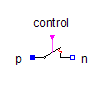Current Quasi RMS Sensor - MapleSim Help

Quasistationary Multiphase Current RMS Sensor

Continuous quasi current RMS sensor for multiphase systemDescription The Quasistationary Multiphase Current RMS Sensor (or Current Quasi RMS Sensor) component outputs the quasi RMS current of a quasistationary multiphase circuit.Equations $i={i}_{p}=-{i}_{n}$ $v={v}_{p}-{v}_{n}$ $I=\sqrt{\frac{1}{m}\sum _{k=1}^{m}{i}_{k}^{2}}$ $\mathrm{\omega }=\frac{d{\mathrm{\gamma }}_{p}}{\mathrm{dt}}$Connections

 Name Description Modelica ID ${\mathrm{plug}}_{p}$ Positive quasistationary multiphase plug plug_p ${\mathrm{plug}}_{n}$ Negative quasistationary multiphase plug plug_n $I$ Continuous quasi average RMS of current IParameters

 Name Default Units Description Modelica ID $m$ $3$ Number of phases mModelica Standard Library The component described in this topic is from the Modelica Standard Library. To view the original documentation, which includes author and copyright information, click here.